Courses

Differences - Digital Signal Processing Notes | Study Digital Signal Processing - Electrical Engineering (EE)

Electrical Engineering (EE): Differences - Digital Signal Processing Notes | Study Digital Signal Processing - Electrical Engineering (EE)

The document Differences - Digital Signal Processing Notes | Study Digital Signal Processing - Electrical Engineering (EE) is a part of the Electrical Engineering (EE) Course Digital Signal Processing.
All you need of Electrical Engineering (EE) at this link: Electrical Engineering (EE)

Differances

UNIT-V

 Non Recursive filters Recursive filters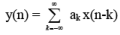for causal system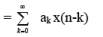For causal i/p sequence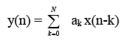It gives FIR o/p. All zero filter. Always stable.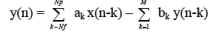for causal system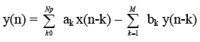It gives IIR o/p but not always.Ex: y(n) = x(n) – x(n-3) + y(n-1)General TF : H(z) =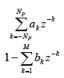bk = 0 for Non RecursiveNf= 0 for causal system

 FIR filters IIR filters 1. Linear phase no phase distortion. Linear phase, phase distortion. 2. Used in speech processing, data transmission & correlation processing Graphic equalizers for digital audio, tone generators filters for digital telephone 3. Realized non recursively. Realized recursively. 4. stable Stable or unstable.H(n) = an u(n)   a<1 stable=  0          a>1 unstable 5. filter order is more Less 6. more co-efficient storage Less storage 7. Quantization noise due to finite precision arithmetic can be made negligible Quantization noise 8. Co-efficient accuracy problem is More less severe 9. used in multirate DSP (variable sampling rate)

The document Differences - Digital Signal Processing Notes | Study Digital Signal Processing - Electrical Engineering (EE) is a part of the Electrical Engineering (EE) Course Digital Signal Processing.
All you need of Electrical Engineering (EE) at this link: Electrical Engineering (EE)Use Code STAYHOME200 and get INR 200 additional OFF Use Coupon Code

Top Courses for Electrical Engineering (EE)Digital Signal Processing

3 videos|50 docs|54 tests

Top Courses for Electrical Engineering (EE)Track your progress, build streaks, highlight & save important lessons and more!

,

,

,

,

,

,

,

,

,

,

,

,

,

,

,

,

,

,

,

,

,

;